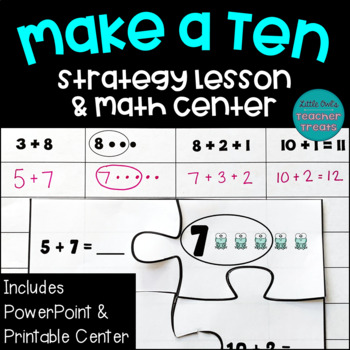# Make a Ten Addition Strategy Lesson and Math Center | Make 10 to Add Activities

Rated 5 out of 5, based on 1 reviews
1 Rating;
1st - 2nd
Subjects
Resource Type
Standards
Formats Included
• Zip
Pages
45 pages
Report this resource to TPT
##### Also included in
1. Are your first and second grade students struggling to understand the Make Ten addition strategy? This easy to use lesson, math center, and set of worksheets will teach and reinforce the make a ten to add concept. This bundle includes 1) a PowerPoint lesson, 2) printable math puzzles, and 3) 22 work
Price \$4.00Original Price \$5.00Save \$1.00

### Description

Are your first and second grade students struggling to understand the Make Ten addition strategy? This easy to use lesson and math center will teach and reinforce the make a ten to add concept. This resource includes a PowerPoint lesson and printable math puzzles that will have your students using dots to make ten, writing new equations, and solving addition problems. Perfect for 1st and 2nd grade whole group, small group, centers, and intervention!

Please see the preview for an up-close look! This lesson simplifies the "make a ten" strategy and teaches this concept in a straightforward, concrete way. The following materials are included:

1) PowerPoint lesson for the "make a ten to add" strategy. Students learn to write the larger number in the equation, draw dots for the smaller number, and compose a ten. Then they write new equations using the ten and the leftover dots. The lesson introduces the strategy, provides guided practice, and includes 5 problems for independent practice.

2) 24 "make a ten" puzzle cards. There are 12 puzzles with 2 pieces, and 12 puzzles with 4 pieces. This allows you to scaffold and differentiate your instruction.

3) Record sheets. There are 3 handouts- 1 for use during the lesson, and 2 for use with the puzzles.

This activity is also available in the following bundle:

Make a Ten Addition Strategy Lesson and Center Bundle

For more Make a Ten practice, try this free resource:

Free Make a Ten Addition Strategy Worksheets

_____________________________

*** Looking for more math resources? Sample my work with these FREEBIES:

Freebie for Set 1: Good Clues, Bad Clues

Freebie for Set 2: Zoo Break

Freebie for Set 3: Put 'Em to Bed - for Google Slides(TM) & PowerPoint(TM)

Freebie for Set 4: To the Rescue

Freebie for Set 6: Fill the Nest

Freebie for Set 7: Super Star

Freebie for Set 8: New Tools, Broken Tools

Freebie for Set 9: Feeding Time

Bonus Freebie: Monster Doubles- for Google Slides(TM) & PowerPoint(TM)

Bonus Freebie: Penguin Place Value- for Google Slides(TM) & PowerPoint(TM)

*** Follow Little Owl's Teacher Treats on TPT for frequent updates, including a "First of the Month" freebie!

Total Pages
45 pages
N/A
Teaching Duration
N/A
Report this resource to TPT
Reported resources will be reviewed by our team. Report this resource to let us know if this resource violates TPT’s content guidelines.

### Standards

to see state-specific standards (only available in the US).
Add and subtract within 20, demonstrating fluency for addition and subtraction within 10. Use strategies such as counting on; making ten (e.g., 8 + 6 = 8 + 2 + 4 = 10 + 4 = 14); decomposing a number leading to a ten (e.g., 13 - 4 = 13 - 3 - 1 = 10 - 1 = 9); using the relationship between addition and subtraction (e.g., knowing that 8 + 4 = 12, one knows 12 - 8 = 4); and creating equivalent but easier or known sums (e.g., adding 6 + 7 by creating the known equivalent 6 + 6 + 1 = 12 + 1 = 13).